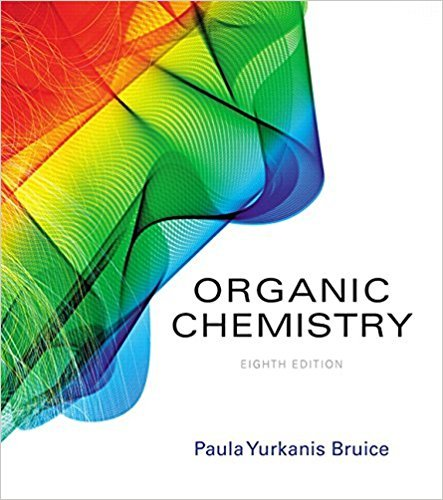×
×

# Under what circumstances can you assume that the less stable of two compounds is theISBN: 9780134042282 482

## Solution for problem 11 Chapter 7.5

Organic Chemistry | 8th Edition

• Textbook Solutions
• 2901 Step-by-step solutions solved by professors and subject experts
• Get 24/7 help from StudySoup virtual teaching assistantsOrganic Chemistry | 8th Edition

4 5 1 294 Reviews
21
1
Problem 11

Under what circumstances can you assume that the less stable of two compounds is the more reactivecompound?

Step-by-Step Solution:
Step 1 of 3

General Chemistry 2 Notes:  Molality: Moles of solute/ kg of solvent  Parts by mass: mass of solute/ mass of solution  Parts by volume: volume of solute/ volume of solution  Mole fraction: moles of solute/ mol of solute + mol of solvent  Parts per million: (mass of solute/ total mass of solution) x 10 ^6 ­ These are all important equations that will need...

Step 2 of 3

Step 3 of 3

##### ISBN: 9780134042282

Unlock Textbook Solution